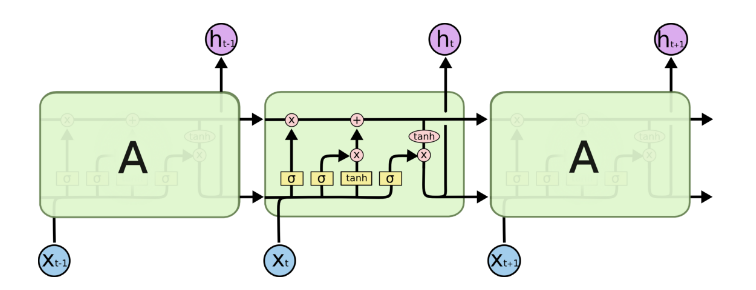torch.nn.LSTM(*args, kwargs)**

• 参数
input_size
hidden_size
num_layers
bias
batch_first
dropout
bidirectional
• 输入
input (seq_len, batch, input_size)
h_0 (num_layers * num_directions, batch, hidden_size)
c_0 (num_layers * num_directions, batch, hidden_size)
• 输出
output (seq_len, batch, num_directions * hidden_size)
h_n (num_layers * num_directions, batch, hidden_size)
c_n (num_layers * num_directions, batch, hidden_size)

## pytorch的LSTM

1、torch.nn.LSTMCell(input_size, hidden_size, bias=True)

2、torch.nn.LSTM(*args, kwargs)**

（感觉说起来没那么简单，所以加了个小标题。）

torch.nn.utils.rnn.pack_sequence(sequences, enforce_sorted=True)

``````import torch as t

a = t.tensor([[1,2,3],[6,0,0],[4,5,0]]) #(batch_size, max_length)
lengths = t.tensor([3,1,2])

# 排序
a_lengths, idx = lengths.sort(0, descending=True)
_, un_idx = t.sort(idx, dim=0)
a = a[idx]

# 定义层
lstm = t.nn.LSTM(input_size=2, hidden_size=4, batch_first=True)

a_input = emb(a)
packed_out, _ = lstm(a_packed_input)
``````# b是另一个batch